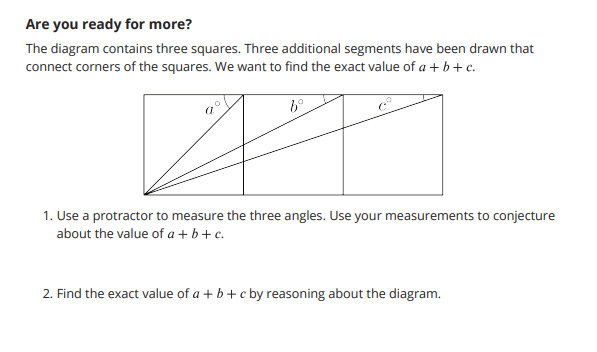PSABM6PH - Student Facing Task---Acc7.3 Lesson 13 Using Equations to Solve for Unknown Angles (7.EE.B.4, 7.G.B.5)
Part A)

Tyler thinks that this figure has enough information to figure out the values of a and b.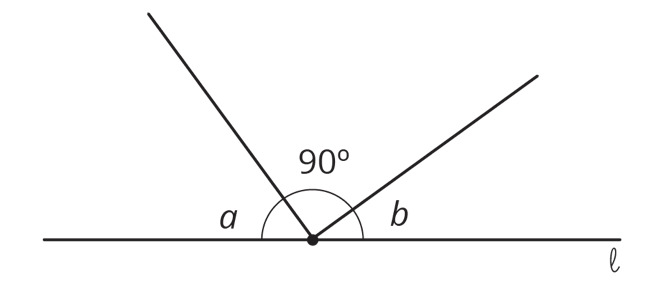Do you agree?

Select one:
Part B)

Part A)

Elena and Diego each wrote equations to represent these diagrams. For each diagram, decide which equation you agree with, and solve it. You can assume that angles that look like right angles are indeed right angles.

1. Elena: 35 Diego: 35 =180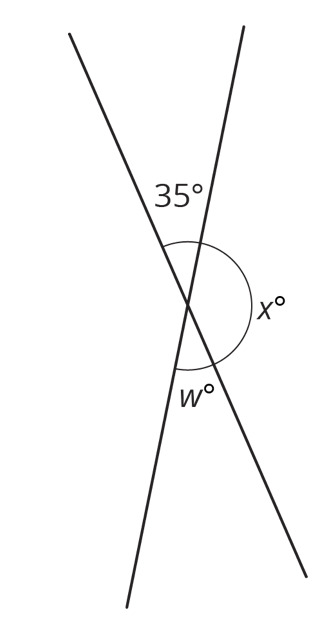Select one:
Part B)

Solve Diego's equation: 35 =180

x = ________

Type your answer below as a number (example: 5, 3.1, 4 1/2, or 3/2):
Part C)

2. Elena: 35 41 180 Diego: 35 180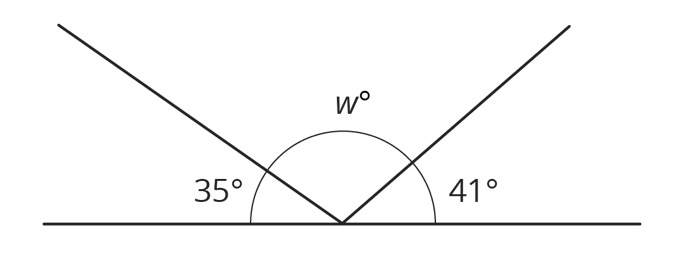Select one:
Part D)

Solve Elena's equation: 341 180

w = _________

Type your answer below as a number (example: 5, 3.1, 4 1/2, or 3/2):
Part E)

3. Elena: 35 90 Diego: 2w 35 90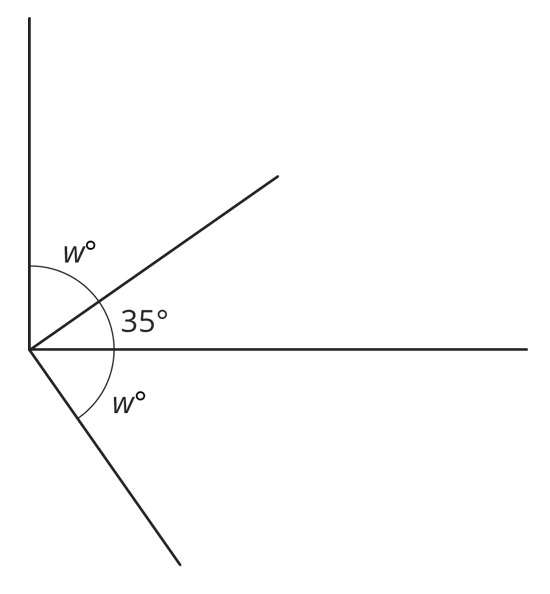Select one:
Part F)

Solve Elena's equation: 35 90

w = _________

Type your answer below as a number (example: 5, 3.1, 4 1/2, or 3/2):
Part G)

4. Elena: 235 90 Diego: 35 90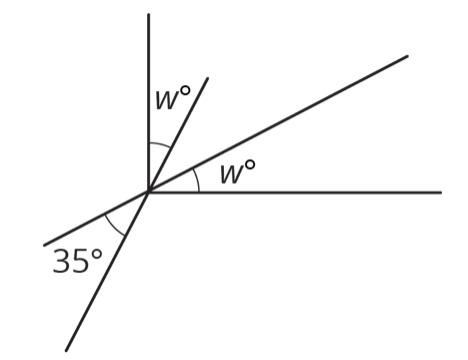Select one:
Part H)

Solve Elena's equation: 235 90

w = _________

235 90

Type your answer below as a number (example: 5, 3.1, 4 1/2, or 3/2):
Part I)

5. Elena: 148 180 Diego: 90 148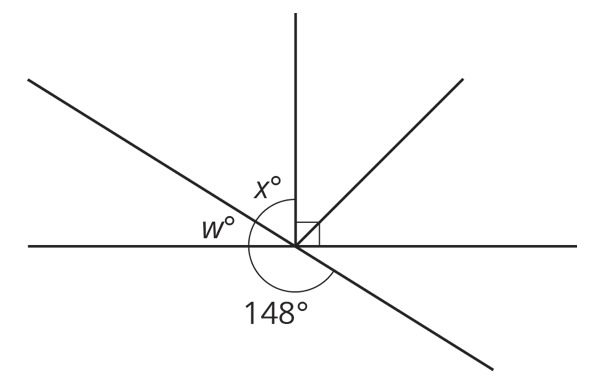Select one:
Part J)

Solve Elena's equation: 148 180

w = _________

Type your answer below as a number (example: 5, 3.1, 4 1/2, or 3/2):
Part K)

Solve Diego's equation: 90 148

x = ________

Type your answer below as a number (example: 5, 3.1, 4 1/2, or 3/2):
Part A)

Find the unknown angle measures.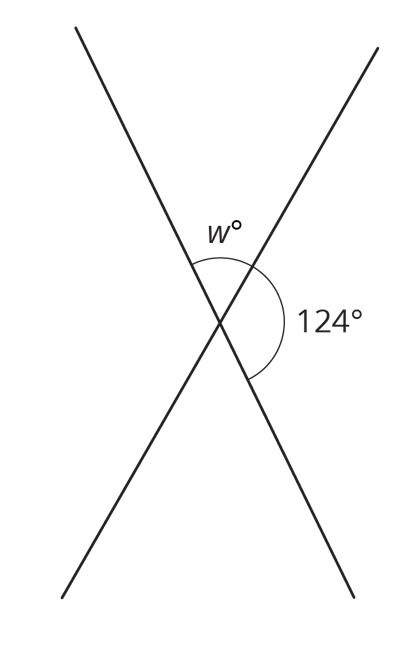w = _______ o

Type your answer below as a number (example: 5, 3.1, 4 1/2, or 3/2):
Part B)

Part C)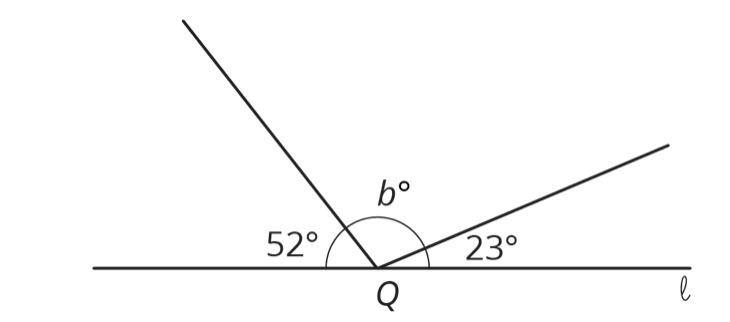b = _______ o

Type your answer below as a number (example: 5, 3.1, 4 1/2, or 3/2):
Part D)

Part E)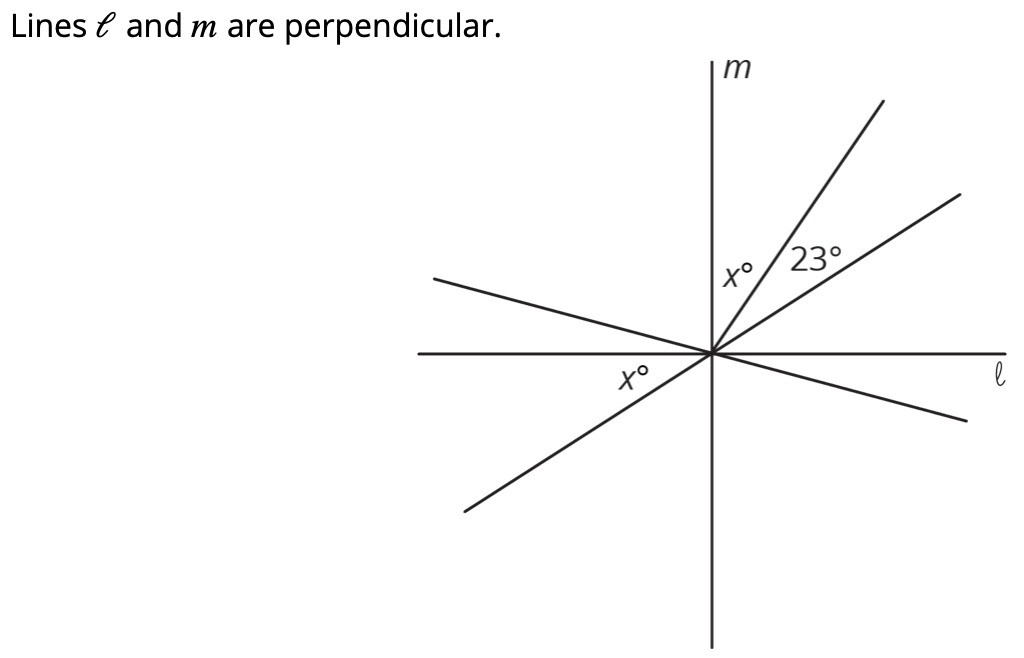x = _______ o

Type your answer below as a number (example: 5, 3.1, 4 1/2, or 3/2):
Part F)

Part G)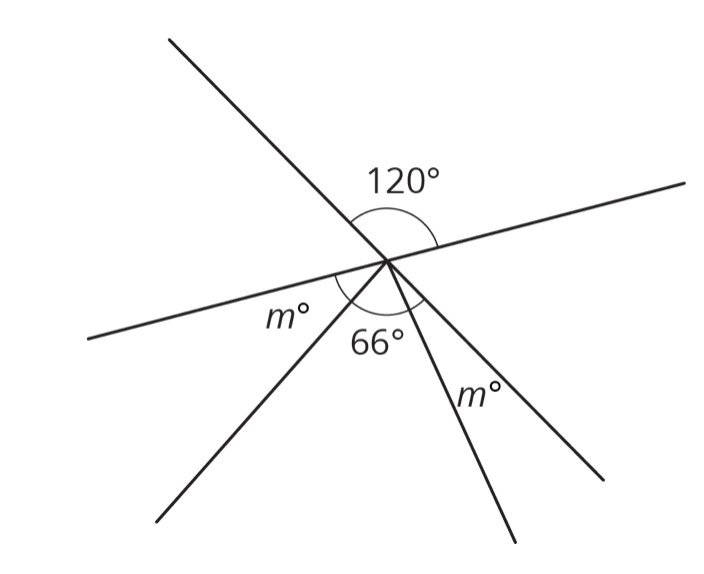m = _______ o

Type your answer below as a number (example: 5, 3.1, 4 1/2, or 3/2):
Part H)